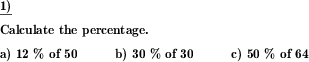Custom math worksheets at your fingertipsDetails for problem "Percentage calculation"

Quickname: 6219

Elementary School, Primary School, Junior High School, Middle School, High School, Senior High School.

Summary

Calculate percentage from base value and rate.

ExampleDescription

A series of percentage calculation problems is given.

The base value is given, which is within a choosable number range. The base value may be a whole number, or a decimal fraction. For the decimal fraction case, the number of decimal places is selectable.

The rate is in the 0 to 100 percent range. A raster of 0.1%, 1%, 5% and 10% can be selected for the rate.

The number of problems is selectable.

Download free printable worksheets for this math problem here. The worksheet contains the problems only, the solution sheet includes the answers. Just click on the respective link.

•Worksheet 1Solution sheet with answers
•Worksheet 2Solution sheet with answers
•Worksheet 3Solution sheet with answers

If you can not see the solution sheets for download, they may be filtered out by an ad blocker that you may have installed. If this is the case, please allow ads for this page and reload the page. The solution sheets will then reappear.

• Do these sample worksheets do not really fit?
• Do you need more math worksheets, with a different level of difficulty?
• Would you like to combine different problems on a worksheet and adjust them to your needs?
• As a teacher, you can put together your own worksheets using the automatically generated math problems provided.
With a free initial credit, you can start creating your own math worksheets in a few minutes.

You can try it for free! Register here, to create custom worksheets now!

Customization options for this problem

Parameter
Possible values
Number of problems
1, 2, 3, 4, 5, 6, 7, 8, 9, 10
Number range
20, 50, 100, 200, 500, 1000, 10000, 100000, 1000000, 10000000
Decimal places
0, 1, 2, 3
Rate raster
0.1%, 1%, 5%, 10%

Similar problems

Remark
Description
Similar, but calculate the base value
Percent rate and percentage are given, calculate the rate and fill in the empty box.
Mixed questions asking for base, rate or percentage
In a percentage term, one of the base, percentage, or percent rate has to be filled in.
Similar, but calculate the rate
Base value and percentage are given, calculate the rate and fill in the empty box.Deutsche Version dieser Aufgabe
These informational pages with samples describe math problems that can be combined on custom math worksheets with solutions for home and K-12 school use.
Deutsche Seiten
×